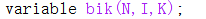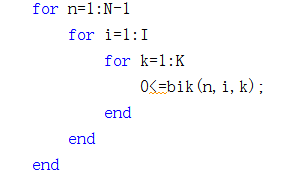# Why is my limit bik>=0, the optimization result of bik will appear -0.0000 (mosek)

The following is my definition, bik is a three-dimensional variableHere is some limit I have on this variableThe optimized result is

There will be a value of -0.000, but since I will also use this variable as a parameter below, since -0.0000 will cause a change in convexity in the optimization below, such as from convex to non-convex. But I hope the value of bik is strictly greater than or equal to 0, how to solve it

The solver I use is mosek

set it zero by hand…

thank you very much！